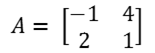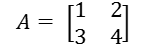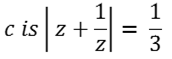MORE IN Applied Mathematics 4
MU Computer Engineering (Semester 4)
Applied Mathematics 4
May 2012
Total marks: --
Total time: --
INSTRUCTIONS
(1) Assume appropriate data and state your reasons
(2) Marks are given to the right of every question
(3) Draw neat diagrams wherever necessary

1 (a) Construct dual penalty of the following LPP:
Max z = 8x1 + 3x2
Subject to x1 - 6x2 ≥ 2
5x1 + 7x2 = -4; x1, x2 ≥ 0
5 M
1 (b) Find the orthogonal tracjectory of the family of curves given by:
2x - x3 + 3xy2 = a
5 M
1 (c) Evaluate ∫{(1/z)dz}, z=C; where C is the upper half of | z |= 1.
5 M
1 (d) Show that every skew-hermitian matrix can be expressed in the form P+iQ where P is real skew-symmetric and Q is real Symmetric matrix.
5 M

2 (a) Determine the analytic function f(z) = - r3sin 3 Θ.
6 M
2 (b) Show that A = is derogatory.6 M
2 (c) Use simplex method to solve the LPP
Max z = 3x1 + 5x2 + 4x3 subject to the constraints:
2x1 + 3x2 ≤ 8, 2x2 + 5x3 ≤ 10
3x1 + 2x2 + 4x1 ≤ 15,
x1, x2, x3 − 0
8 M

3 (a) Evaluate -∞ {(x2 - x + 2)/(x4 + 10x2 + 9) dx}; using contour integration.
6 M
3 (b) Find the eigen values and eigen vectors of A3 where A =6 M
3 (c) Use penalty method (Big M) to solve Mini z = 4x + y subject to
3x + y = 3,
4x + 3y ≥ 6,
x + 2y ≤ 4,
x, y ≥ 0
8 M

4 (a) If w = f(z) then prove that
(dw/dz) = (cosθ - isinθ) (∂w/∂r)
6 M
4 (b) If A is orthogonal, find a,b,c. Also find A-1.6 M
4 (c) Use dual simplex method to solve the LPP.
Minimize z = 6x1 + 7x2 + 3x3 + 5x4;
subject to 5x1 + 6x2 - 3x3 + 4x4 ≥ 12
x2 + 5x3 - 6x4 ≥ 10
2x1 + 5x2 + x3 + x4 ≥ 8;
x1, x2, x3, x4 ≥ 0
8 M

5 (a) Prove that 3tan A = Atan 3, where:6 M
5 (b) Find the image of the line y - x + 1 = 0 under the transformation w = 1/z. Also find the image of the line y = x under the same transformation. Draw rough sketches.
6 M
5 (c) Solve the NLPP using the method of Lagrangian Multipliers.
Minimize z = x12 + x22 + x32;
subject to x1 + x2 + 3x3 = 2, 5x1 + 2x2 + x3 = 5,
x1, x2, x3 ≥ 0
8 M

6 (a) Verify caley-hamiton theorem for A and hence find
A-1
A3 - 5A2.
where,6 M
6 (b) Find the bilinear transformation that maps the points ∞, i, 0 onto the points 0, i, ∞. Find the fixed points.
6 M
6 (c) State and prove Cauchy s integral theorem and hence evaluate,
where,8 M

7 (a) Obtain two Laurent s series for {[(z-2)(z+2)] / [(z+1)(z+4)]}
6 M
7 (b) Evaluate 0{(cos 2θ) / (1 - 2acosθ + a2)) dθ}, using residues.
6 M
7 (c) Solve the following N.L.P.P. using Kuhn-Tucker conditions.
Minimize z = 7x12 + 5x22 - 6x1 subject to
x1 + 2x2 ≤ 10
x1 + 3 x2 ≤ 9
x1, x1 ≥ 0.
8 M

More question papers from Applied Mathematics 4